Custom SearchIMAGINARY UNIT To reduce the problem of imaginary numbers to its simplest terms, we proceed as far as possible using ordinary numbers in the solution. Thus, we may write asa productLikewise,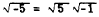Also,Thus, the problem of giving meaning to the square root of any negative number reduces to that of finding a meaning for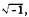The square root of minus 1 is designated i by mathematicians. When it appears with a coefficient, the symbol i is written last unless the coefficient is in radical form. This convention is illustrated in the following examples:The symbol i stands for the imaginary unit. An imaginary number is any real multiple, positive or negative, of i. For example, -7i, +7i, i, and bi are all imaginary numbers. In electrical formulas the letter i denotes current. To avoid confusion, electronic technicians use the letter j to indicateand call it "operator j." The name "imaginary" should be thought of as a technical mathematical term of convenience. Such numbers have a very real purpose in the physical sense. Also it can be shown that ordinary mathematical operations such as addition, multiplication, and so forth, may be performed in exactly the same way as for the so-called real numbers. Practice problems. Express each of the following as some real number times i:Powers of the Imaginary Unit  The following examples illustrate the results of raising the imaginary unit to various powers: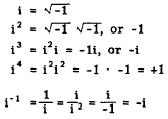We see from these examples that an even power of i is a real number equal to +1 or -1. Every odd power of i is imaginary and equal to i or -i. Thus, all powers of i reduce to one of the following four quantities:, -1, -, or +1. GRAPHICAL REPRESENTATION Figure 15-1 shows the real numbers represented along a straight line, the positive numbers extending from zero to the right for an infinite distance, and the negative numbers extending to the left of zero for an infinite distance. Every point on this line corresponds to a real number, and there are no gaps between them. It follows that there is no possibility of representing imaginary numbers on this line. Earlier, we noted that certain signs could be used as operators. The plus sign could stand for the operation of addition. The minus sign could stand for the operation of subtraction. Likewise, it is easy to explain the imaginary number i graphically as an operator indicating a certain operation is to be performed on the number of which it is the coefficient. If we graphically represent the length, n, on the number line pictured in figure 15-2 (A), we start at the point 0 and measure to the right (positive direction) a distance representing n units. If we multiply n by -1, we may represent the result -n by measuring from 0 in a negative direction a distance equal to n units. Graphically, multiplying a real number by -1 is equivalent to rotating the line that represents the number about the point 0 through 180° so that the new position of n is in the opposite direction and a distance n units from 0. In this case we may think of -1 as the operator that rotates n through two right angles to its new position (fig. 15-2 (B)). As we have shown, i2 = -1. Therefore, we have really multiplied n by i2, or i x i. In other words, multiplying by -1 is the same as multiplying by i twice in succession. Logically, if we multiplied n by i just once, the line n would be rotated only half as much as before-that is, through only one right angle, or 90°. The new segment ni would be measured in a direction 90" from the line n. Thus, i is an operator that rotates a number through one right angle. (See fig. 15-3.) We have shown previously that a positive number may have two real square roots, one positive and one negative. For example,= ±3.Figure 15-2.-Graphical multiplication by -1 and by operator i2 .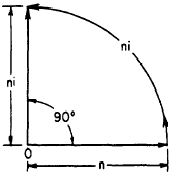Figure 15-3. -Graphical multiplication by operator i. We also saw that an imaginary number may have two roots. For example,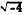is equal to ±2i. When the operator -1 graphically rotates a number, it may do so in a counterclockwise or a clockwise direction. Likewise, the operator i may graphically rotate a number in either direction. This fact gives meaning to numbers such as ±2i. It has been agreed that a number multiplied by +i is to be rotated 90° in a counterclockwise direction. A number multiplied by -i is to be rotated 90° in a clockwise direction. In figure 15-4, +2i is represented by rotating the line that represents the positive real number 2 through 90° in a counterclockwise direction. It follows that -2i is represented by rotating the line that represents the positive real number 2 through 90° in a clockwise direction.Figure 15-4.-Graphical representation of ± 2i. In figure 15-5, notice that the idea of i as an operator agrees with the concept advanced concerning the powers of i. Thus, i rotates a number through 90°; 1 2 or -1 rotates the number through 180°, and the number is real and negative; i3 rotates the number through 270°, which has the same effect as -i; and i4 rotates the number through 360°, and the number is once again positive and real.